## Introduction

The popgraph package is designed to take multivariate data and construct a Population Graph (Dyer & Nason 2004). This is a graph-theoretic interpretation of genetic covariance and serves as a tool for understanding underlying evolutionary history for a set of populations.

These routines were originally in the gstudio package but were excised out for simplicity. This analysis is not limited solely to genetic data and can be used generally for many types of analyses. As such, I pulled this out of the genetic package and allow it to remain on its own. To get your data using gstudio with genotypes and such into a format for this package, translate the genotypes into their multivariate format as:

``data <- as.matrix( my_genetic_data )``

For more information on this, see the documentation on the gstudio package (a copy is mirrored at http://dyerlab.bio.vcu.edu/ and a clone of the package can be checked out at https://github.com/dyerlab/gstudio)

## Creating Population Graphs

There are two ways to create a population graph:

1. In this package using the function popgraph() and,
• Via the servers at http://dyerlab.bio.vcu.edu (which use this packages to do the translation) or via GeneticStudio (an older software package)
``require(popgraph)``

### Creating De Novo Graphs

Here we will focus on the former approach as it is native to this package. If you use the latter one, it will produce a *.pgraph file and you can read it in using

``````A <- matrix(0, nrow=5, ncol=5)
A[1,2] <- A[2,3] <- A[1,3] <- A[3,4] <- A[4,5] <- 1
A <- A + t(A)
A``````
``````##      [,1] [,2] [,3] [,4] [,5]
## [1,]    0    1    1    0    0
## [2,]    1    0    1    0    0
## [3,]    1    1    0    1    0
## [4,]    0    0    1    0    1
## [5,]    0    0    0    1    0``````

There is a quick function, `as.popgraph()` that takes either an existing igraphobject or a matrix and turns them into popgraph objects.

``g <- as.popgraph( A )``

There are several options available under the `mode` parameter. We typically use the undirected graph option but the following are also available:

1. `undirected` The connections between nodes are symmetric. This is the default for population graphs as covariance, the quantity the edge is representing is symmetrical.
• `directed` The edges are asymetric.
• `max` or `min` Will take the largest (or smallest) value of the matrix (e.g., \(max(A[i,j], A[j,i])\) or \(min( A[i,j], A[j,i])\) ).
• `upper` or `lower` Uses either the upper or lower element of the matrix.
• `plus` Adds upper and lower values (e.g., \(A[i,j] + A[j,i]\)).

There are many other ways to create igraphobjects de novo but this is the easiest method.

#### Node & Edge Attributes

The underlying structure of an igraphobject allows you to assoicate attributes (e.g., other data) with nodes and edges. Node attributes are accessed using the \(V(graph)\) operator (for vertex) and edge attributes are done via \(E(graph)\). Attributes can be set as well as retrieved using the same mechanisms.

``````V(g)\$name <- c("Olympia","Bellingham","St. Louis","Ames","Richmond")
V(g)\$group <- c("West","West", "Central","Central","East")
V(g)\$color <- "#cca160"
list.vertex.attributes( g )``````
``##  "name"  "group" "color"``
``V(g)\$name``
``##  "Olympia"    "Bellingham" "St. Louis"  "Ames"       "Richmond"``
``E(g)``
``````## Edge sequence:
##
##  Bellingham -- Olympia
##  St. Louis  -- Olympia
##  St. Louis  -- Bellingham
##  Ames       -- St. Louis
##  Richmond   -- Ames``````
``````E(g)\$color <- c("red","red", "red", "blue","dark green")
list.edge.attributes( g )``````
``##  "weight" "color"``

### Adding data to a graph

A population graph is made more informative if you can associate some data with topology. External data may be spatial or ecolgoical data associated with each node. Edge data may be a bit more complicated as it is traversing both spatial and ecolgoical gradients and below we’ll see how to extract particular from rasters using edge crossings.

Included in the popgraph package are some build-in data sets. You can load these into R using the `data()` function as:

``````data(lopho)
class(lopho)``````
``##  "popgraph" "igraph"``
``lopho``
``````## IGRAPH UNW- 21 52 --
## + attr: name (v/c), size (v/n), color (v/c), Region (v/c), weight
##   (e/n)``````

The function `decorate_graph()` allows you to add more information to the graph object by combining data from an external source, in this case a `data.frame` object. Here is an example with some built-in data. The option `stratum` indicates the name of the column that has the node labels in it (which are stored as `V(graph)\$name`).

``````data(baja)
summary(baja)``````
``````##     Region     Population    Latitude      Longitude
##  Baja  :16   BaC    : 1   Min.   :22.9   Min.   :-115
##  Sonora:13   Cabo   : 1   1st Qu.:24.4   1st Qu.:-113
##              CP     : 1   Median :27.9   Median :-112
##              Ctv    : 1   Mean   :27.3   Mean   :-112
##              ELR    : 1   3rd Qu.:29.6   3rd Qu.:-111
##              IC     : 1   Max.   :31.9   Max.   :-109
##              (Other):23``````
``````lopho <- decorate_graph( lopho, baja, stratum="Population")
lopho``````
``````## IGRAPH UNW- 21 52 --
## + attr: name (v/c), size (v/n), color (v/c), Region (v/c),
##   Latitude (v/n), Longitude (v/n), weight (e/n)``````

Each vertex has seveal different types of data associated with it now. We will use this below.

### Plotting a graph using normal plotting methods

One of the main benefits to using R is that you can leverage the mutlitude of other packages to visualize and manipulate your data in interesting and informative ways. Since a `popgraph` is an instance of an igraphelement, we can use the igraphroutines for plotting. Here is an example.

``plot(g)``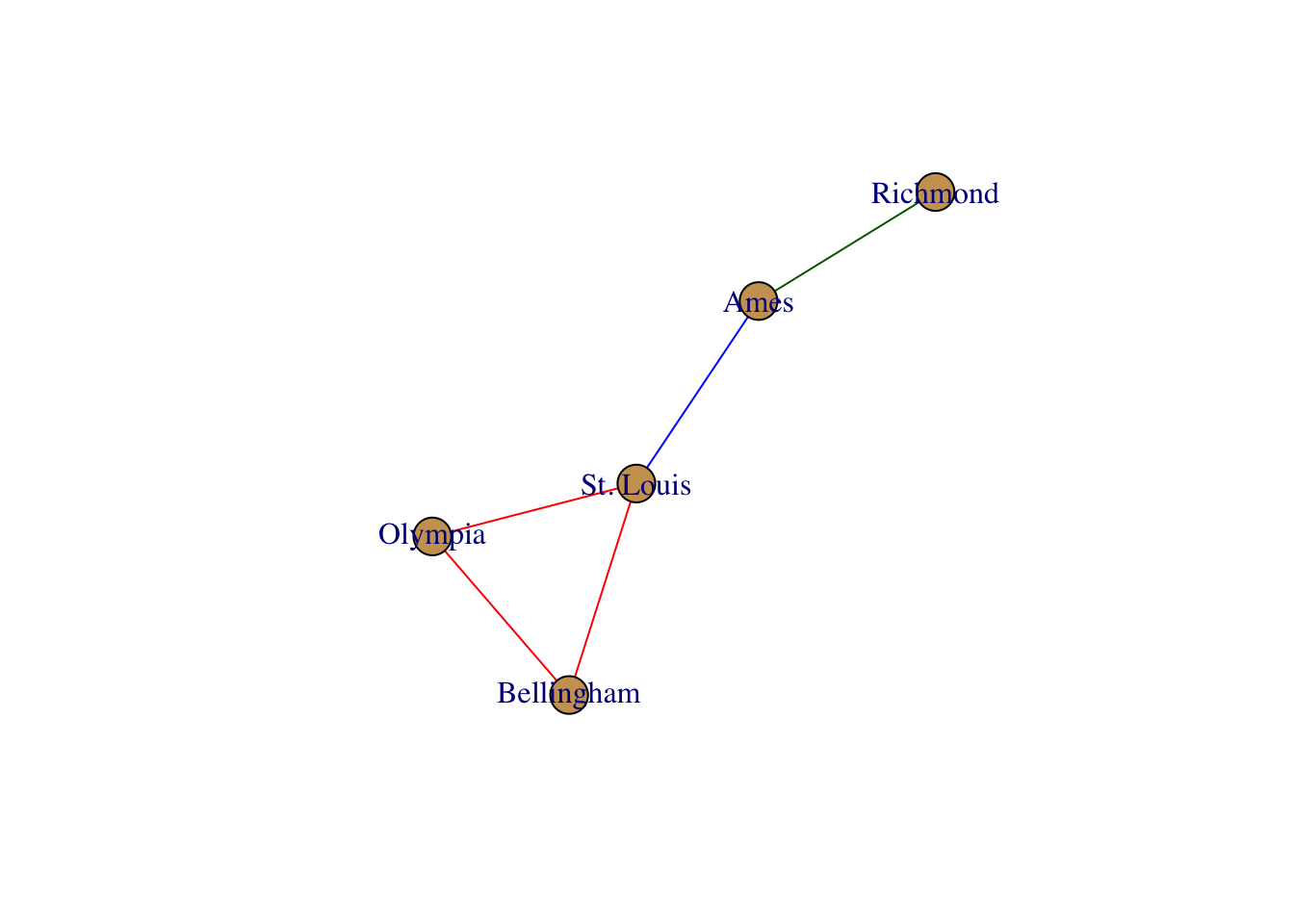There are several different options you can use to manipulate the graphical forms. By default, the plotting routines look for node and edge attributes such as `name` and `color` to plot the output appropriately. There are several additional plotting functions for plotting igraph objects. Here are some examples.

``plot(g, edge.color="black", vertex.label.color="darkred", vertex.color="#cccccc", vertex.label.dist=1)``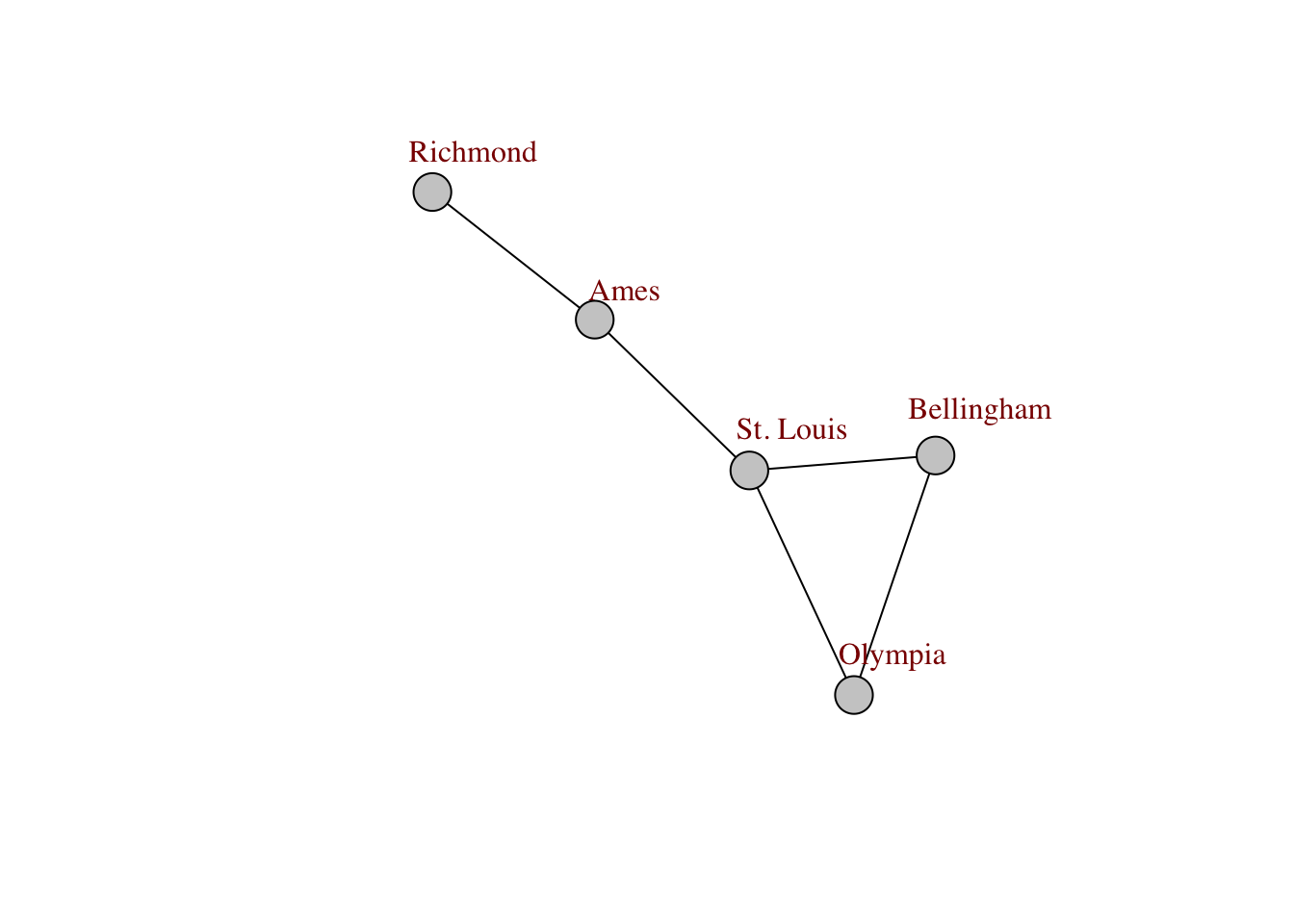``````layout <- layout.circle( g )
plot( g, layout=layout)``````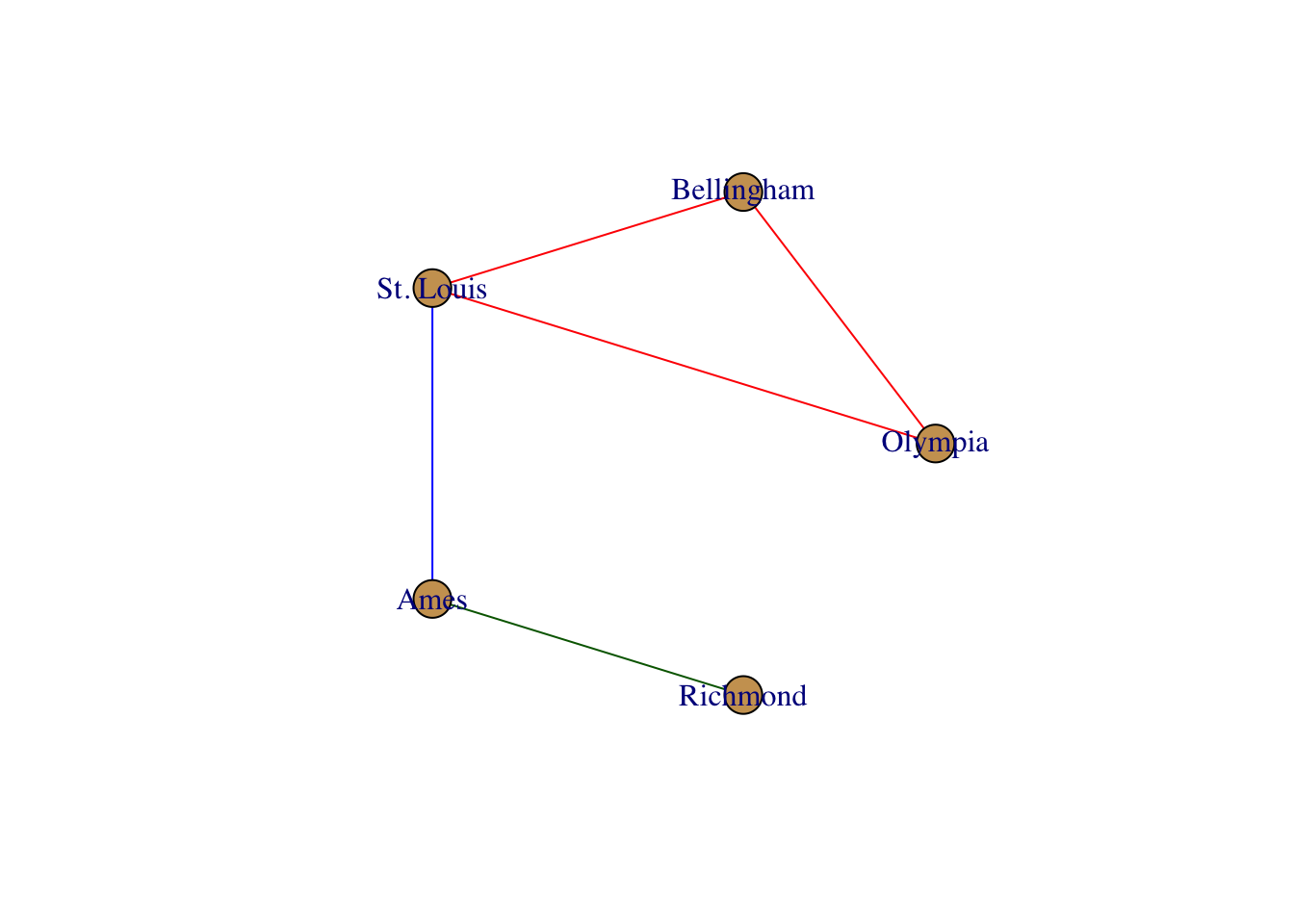``````layout <- layout.fruchterman.reingold( g )
plot( g, layout=layout)``````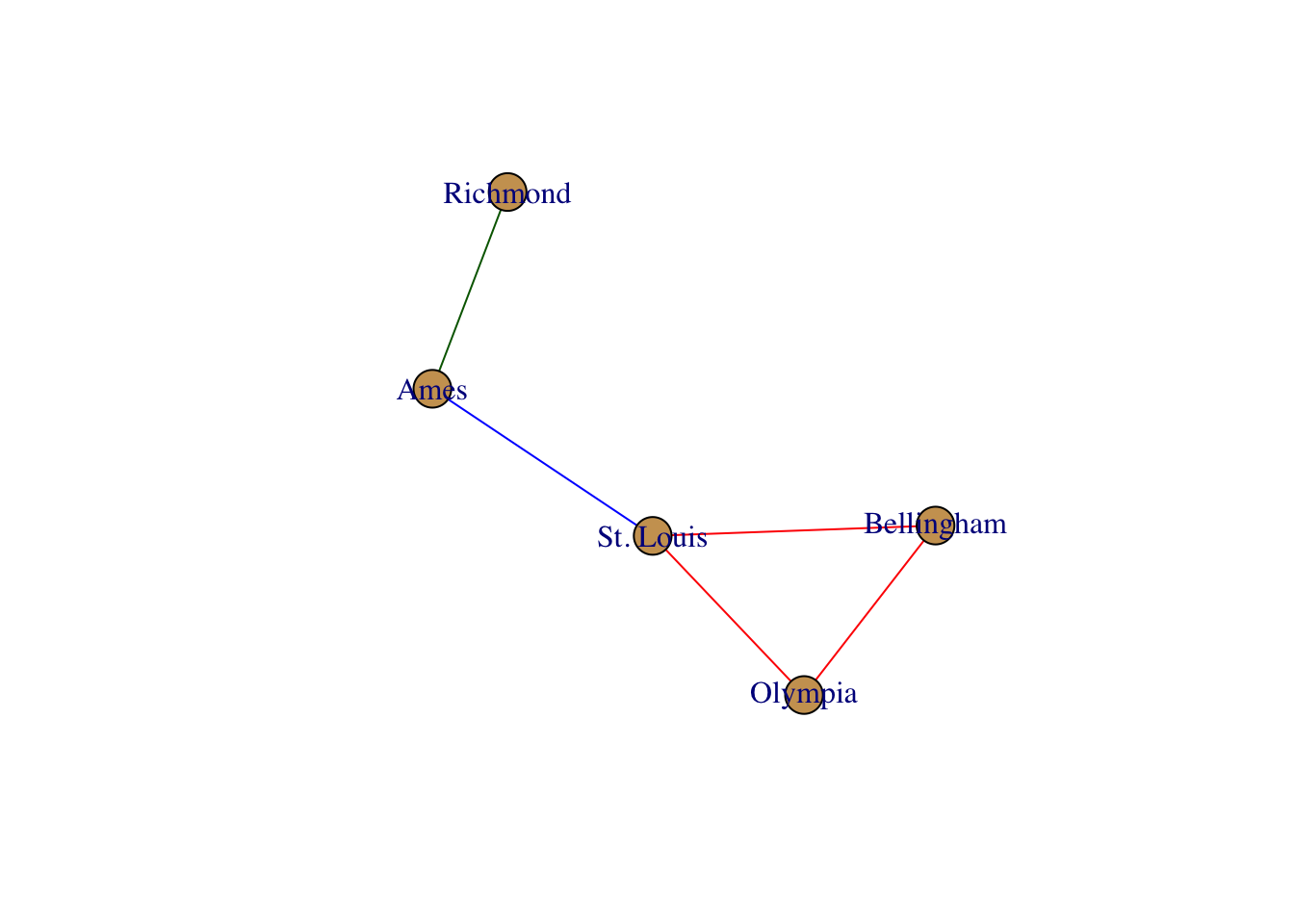### Plotting a graph using ggplot2 routines

The ggplot2 package provides a spectacular plotting environment in an intuitive context and there are now some functions to support the Population Graphs in this context.

If you haven’t used ggplot2 before, it may at first be a bit odd because it deviates from normal plotting approaches where you just shove a bunch of arguments into a single plotting function. In ggplot, you build a graphic in the same way you build a regression equation. A regression equation has an intercept and potentially a bunch of independent terms. This is exactly how ggplot builds plots, by adding togther components.

To specifiy how things look in a plot, you need to specify an aesthetic using the `aes()` funciton. Here is where you supply the variable names you use for coordinate, coloring, shape, etc. For both of the `geom_*set` funcitons, these names must be attributes of either the node or edge sets in the graph itself.

Here is an example using the Lopohcereus graph. We begin by making a `ggplot()` object and then adding to it a `geom_` object. The 5popgraph package comes with two funcitons, one for edges and one for nodes.

``````require(ggplot2)
p <- ggplot()
p <- p + geom_edgeset( aes(x=Longitude,y=Latitude), lopho )
p``````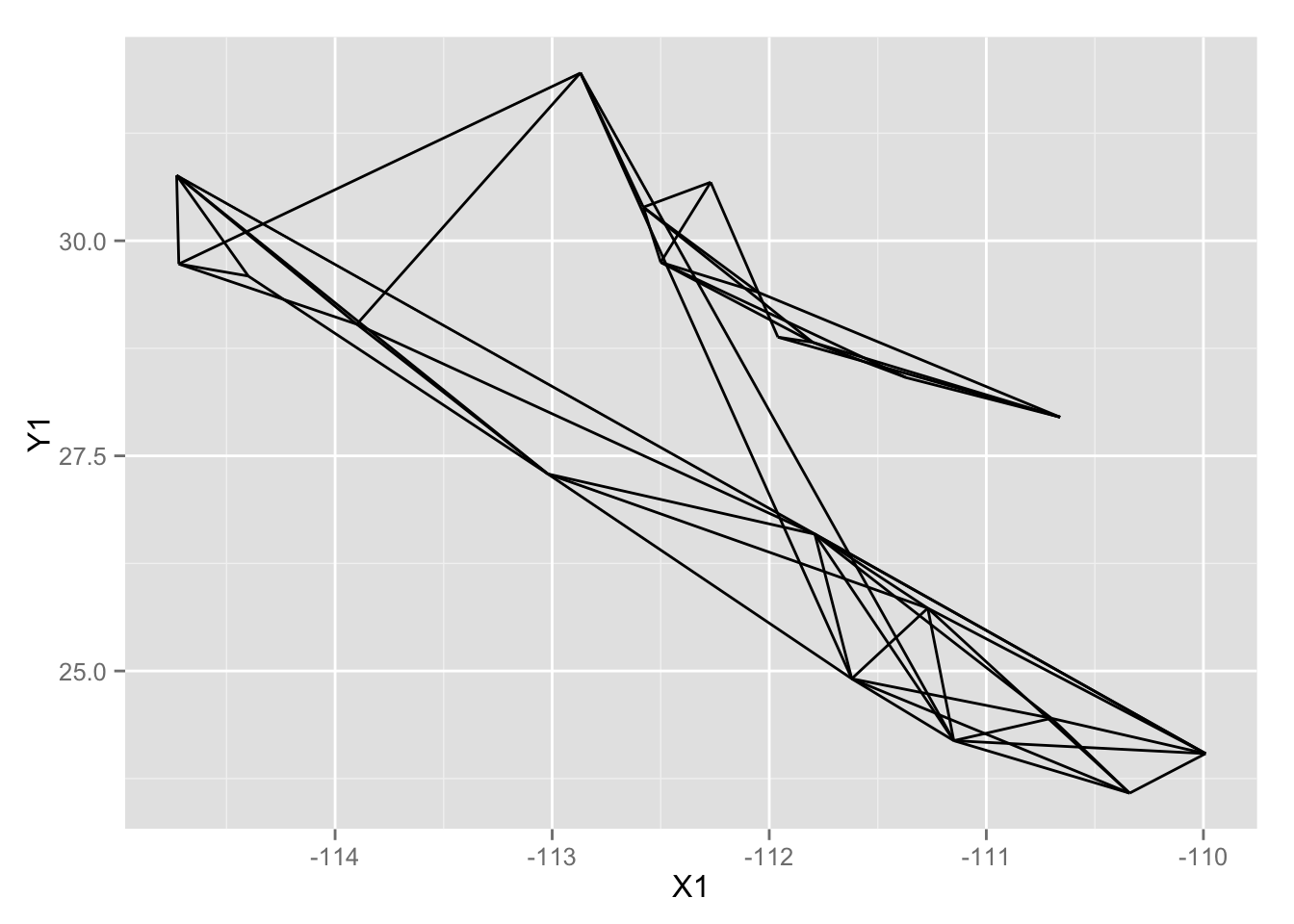I broke up the plotting into several lines to improve readability, it is not necessary to to this in practice though. The addition of additional `geom_` objects to the plot will layer them on top (n.b., I also passed the size=4 option to the plot as the default point size is a bit too small and this is how you could change that).

``````p <- p +  geom_nodeset( aes(x=Longitude, y=Latitude), lopho, size=4)
p``````